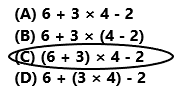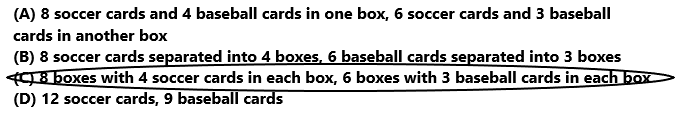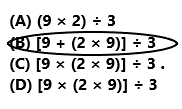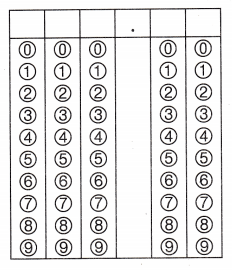Refer to our Texas Go Math Grade 5 Answer Key Pdf to score good marks in the exams. Test yourself by practicing the problems from Texas Go Math Grade 5 Module 7 Assessment Answer Key.

Vocabulary

Choose the best term from the box.

Vocabulary
composite number
numerical expression
prime number

Question 1.
A ___________ is a whole number greater than 1 that has exactly two factors, 1 and itself. (p. 297)
A Prime number is a whole number greater than 1 that has exactly two factors, 1 and itself.

Question 2.
A ____________ is a mathematical phrase that has numbers and operation signs but does not have an equal sign. (p. 303)
A numerical expression is a mathematical phrase that has numbers and operation signs but does not have an equal sign.

Concepts and Skills

Decide if the number Is prime or composite. If it is composite, list the factor pairs. (TEKS 5.4.A)

Question 3.
54
The number 54 is a composite number.
The factor pairs are as below.
1 × 54 = 54
2 × 27 = 54
3 × 18 = 54
6 × 9 = 54
9 × 6 = 54
Explanation:
In Mathematics, composite numbers are numbers that have more than two factors. The number 54 is a composite number. The factors of 54 are 1, 2, 3, 6, 9, 18, 27, 54. The factor pairs of 54 are (1, 54), (2, 27), (3, 18), (6, 9), (9,6).

Question 4.
28
The number 28 is a composite number.
The factor pairs areas below.
1 x 28 = 28
2 x 14 = 28
4 x 7 = 28
7 x 4 = 28
Explanation:
In Mathematics, composite numbers are numbers that have more than two factors. The number 28 is a composite number. The factors of 28 are 1, 2, 4, 7, 14, 28. The factor pairs of 28 are (1, 28), (2, 14), (4, 7), (7,4).

Tell whether the number Is prime or composite. (TEKS 5.4.A)

Question 5.
33
The factors of 33 are 1, 3, 11, 33.
So, the number 33 is a composite number.
Explanation:
A composite number is a number that can be divided evenly by more numbers than 1 and itself. The number 33 can be evenly divided by 1, 3, 11 and 33, with no remainder. Since 33 cannot be divided by just 1 and 33. So, 33 is a composite number.

Question 6.
47
The factors of 47 are 1, 47. So, 47 is a prime number.
Explanation:
47 is a prime number. The number 47 is divisible only by 1 and the number itself. The number 47 is classified as a prime number, because it have exactly two factors.

Question 7.
91
The factors of 91 are 1, 7, 13, 91. So, 91 is a composite number.
Explanation:
A composite number is a number that can be divided evenly by more numbers than 1 and itself. It is the opposite of a prime number. The number 91 can be evenly divided by 1, 7, 13, 91 with no remainder. Since 91 cannot be divided by just 1 and 91. So, it is a composite number.

Question 8.
81
The factors of 81 are 1, 3, 9, 27, 81
So, the number 81 is a composite number.
Explanation:
A composite number is a number that can be divided evenly by more numbers than 1 and itself. It is the opposite of a prime number. The number 81 can be evenly divided by 1, 3, 9, 27 and 81, with no remainder. Since 81 cannot be divided by just 1 and 81. So, it is a composite number.

Simplify the numerical expression. (TEKS 5.4.F)

Question 9.
18 – (8 × 3) ÷ 4
18 – (8 × 3) ÷ 4
18 – 24 ÷ 4
18 – 6
12
Explanation:
The numerical expression is 18 – (8 × 3) ÷ 4. Perform the operations in the parentheses first 18 – 24 ÷ 4. Next perform the division operation 18 – 6. Then perform subtraction operation the difference is 12. The simplified form of given numerical expression is 12.

Question 10.
35 – [(4 × 5) + (2 × 5)]
35 – [(4 × 5) + (2 × 5)]
35 – [20 + 10]
35 – 30
5
Explanation:
The numerical expression using parentheses and brackets is 35 – [(4 × 5) + (2 × 5)]. Perform the operations in the parentheses first 35 – [20 + 10]. Next perform the operations in the brackets 35 – 30. Then perform subtraction operation the difference is 5. The simplified form of given numerical expression is 5.

Question 11.
Students in a math contest are asked to simplify a numerical expression. The correct answer is 34. Which could be the expression? (TEKS 5.4.F)
(A) 6 + 3 × 4 – 2
(B) 6 + 3 × (4 – 2)
(C) (6 + 3) × 4 – 2
(D) 6 + (3 × 4) – 2(6 + 3) × 4 – 2
9 x 4 – 2
36 – 2
34
So, option C is correct.
Explanation:
Students in a math contest are asked to simplify a numerical expression. The correct answer is 34. The numerical expression is (6 + 3) × 4 – 2. The simplified form of numerical expression is 34.

Question 12.
Ana writes the expression (8 × 4) + (6 × 3) to represent the number of cards in her sports card collection. Which could be Ana’s sports card collection? (TEKS 5.4.E)
(A) 8 soccer cards and 4 baseball cards in one box, 6 soccer cards and 3 baseball cards in another box
(B) 8 soccer cards separated into 4 boxes, 6 baseball cards separated into 3 boxes
(C) 8 boxes with 4 soccer cards in each box, 6 boxes with 3 baseball cards in each box
(D) 12 soccer cards, 9 baseball cardsOption C is correct.
Explanation:
Ana writes the expression (8 × 4) + (6 × 3) to represent the number of cards in her sports card collection. Ana’s sports card collection is 8 boxes with 4 soccer cards in each box, 6 boxes with 3 baseball cards in each box.

Question 13.
A florist has 9 daffodils and twice as many tulips. He donates the flowers equally to 3 parks. Which expression represents the number of flowers each park receives? (TEKS 5.4.E)
(A) (9 × 2) ÷ 3
(B) [9 + (2 × 9)] ÷ 3
(C) [9 × (2 × 9)] ÷ 3 .
(D) [9 × (2 × 9)] ÷ 3Option B is correct.
Explanation:
A florist has 9 daffodils and twice as many tulips. He donates the flowers equally to 3 parks. The expression represents the number of flowers each park receives is [9 + (2 × 9)] ÷ 3.

Question 14.
David washes 10 cars and waxes 4 cars every Saturday. He earns $5 for each car he washes and$12 for each car he waxes. How much money does he earn on 3 Saturdays in dollars? Simplify the expression 3 × [(10 × 5) + (4 × 12)] to find the answer. (TEKS 5.4.F)Record your answer and fill in the bubbles on the grid. Be sure to use the correct place value.$294.00 On 3 Saturdays he earns$294.00.
David washes 10 cars and waxes 4 cars every Saturday. He earns $5 for each car he washes and$12 for each car he waxes. The expression is 3 × [(10 × 5) + (4 × 12)]. The simplified form of the expression is $294.00. On 3 Saturdays he earns$294.00.